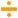# Divide

 Block Group: Math Operations Icon:The Divide block divides its arguments.

Division is calculated in order, top to bottom.

For information on using dataflow blocks, see Dataflow.

For accepted and excluded arguments of Math Operations blocks, see Math Operations.

## Input/Output Properties

The following properties of the Divide block can take input and give output.

• input 0 (number)
• input n (number)

input 0 specifies the initial dividend.

input n specifies a divisor. Division is calculated in order, top to bottom.

## Output Property

The following property of the Divide block can give output but cannot take input.

• output (number)

output returns the result of dividing all of the input values.

## Special Case for the Divide Block

• A divisor of zero causes an “Infinity” error result to be returned.

## Examples

The following image shows two examples of the Divide block. In the leftmost Divide block, ten is divided first by four and then by two, for an output value of 1.25. In the rightmost Divide block, two different data types are used: a number and a string representation of a number.